# Data in Nuclear Engineering

### Kathryn (Katy) Huff$\phi(E,\vec{r},\hat{\Omega},T)$

$\phi(E, x, y, z, \theta, \omega, T)$$\sigma(x, i, E, T)$$\gamma(f, i)$$i$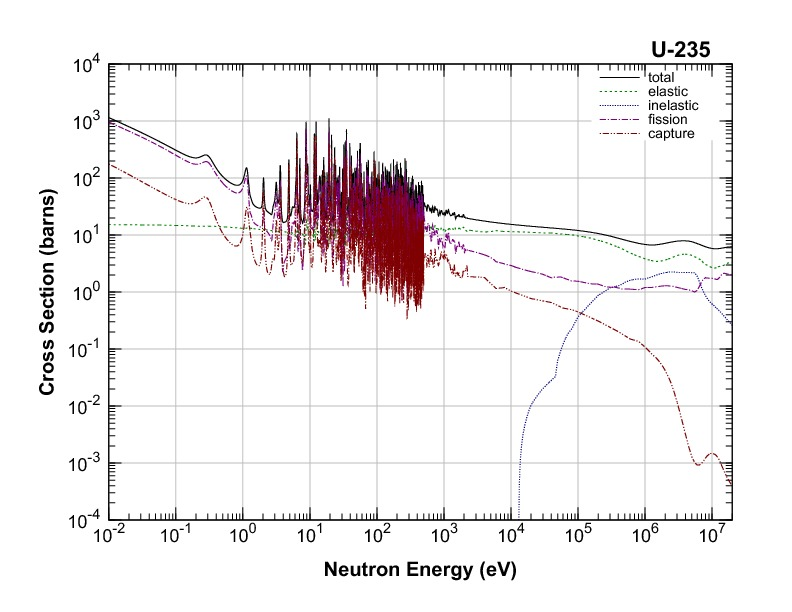$E$nuclear structure

## Evaluated Nuclear Data Sets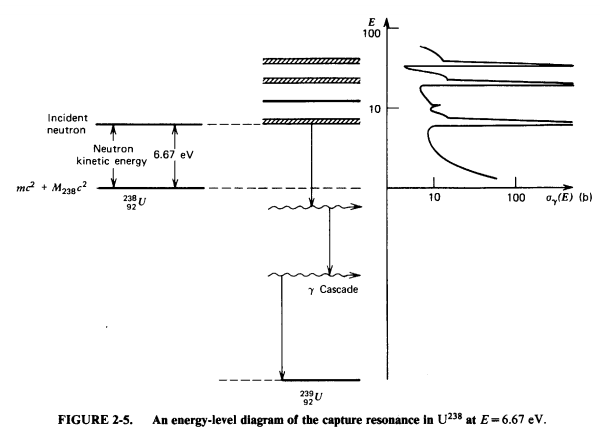$\sigma(x, i, E, T)=$ nuclear structure + experiments

## Experiments## Experiments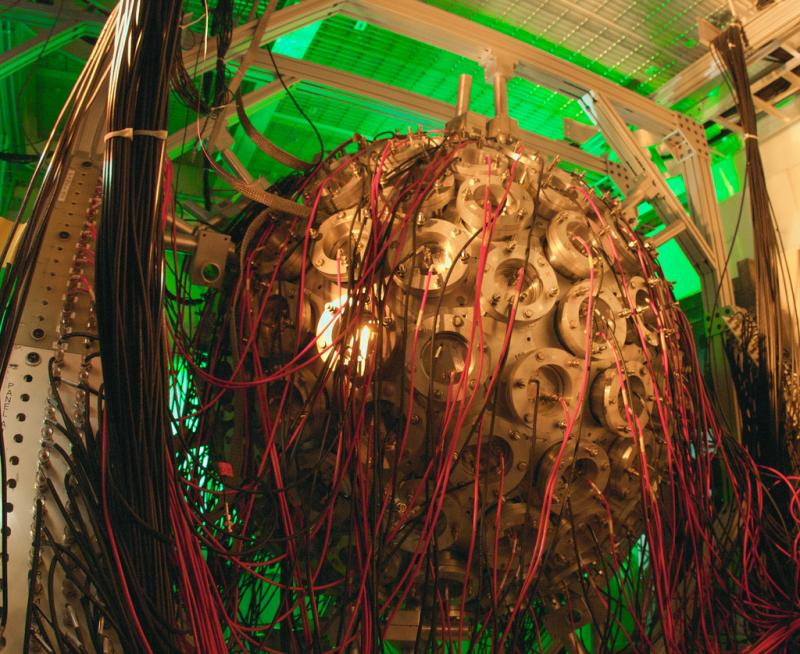## Nuclear Data is for Simulations## Simulation Methods

• Monte Carlo Methods
• Deterministic Methods
• Hybrid Methods
• Other keywords...
• lattice codes
• ray tracing algorithms
• acceleration schemes
• ...

## Application Specific Data Processing

• Energy discretization
• multigroup
• pointwise
• piecewise linear continuous
• Resonance integration
• ...

### MSBR Full Core Simulation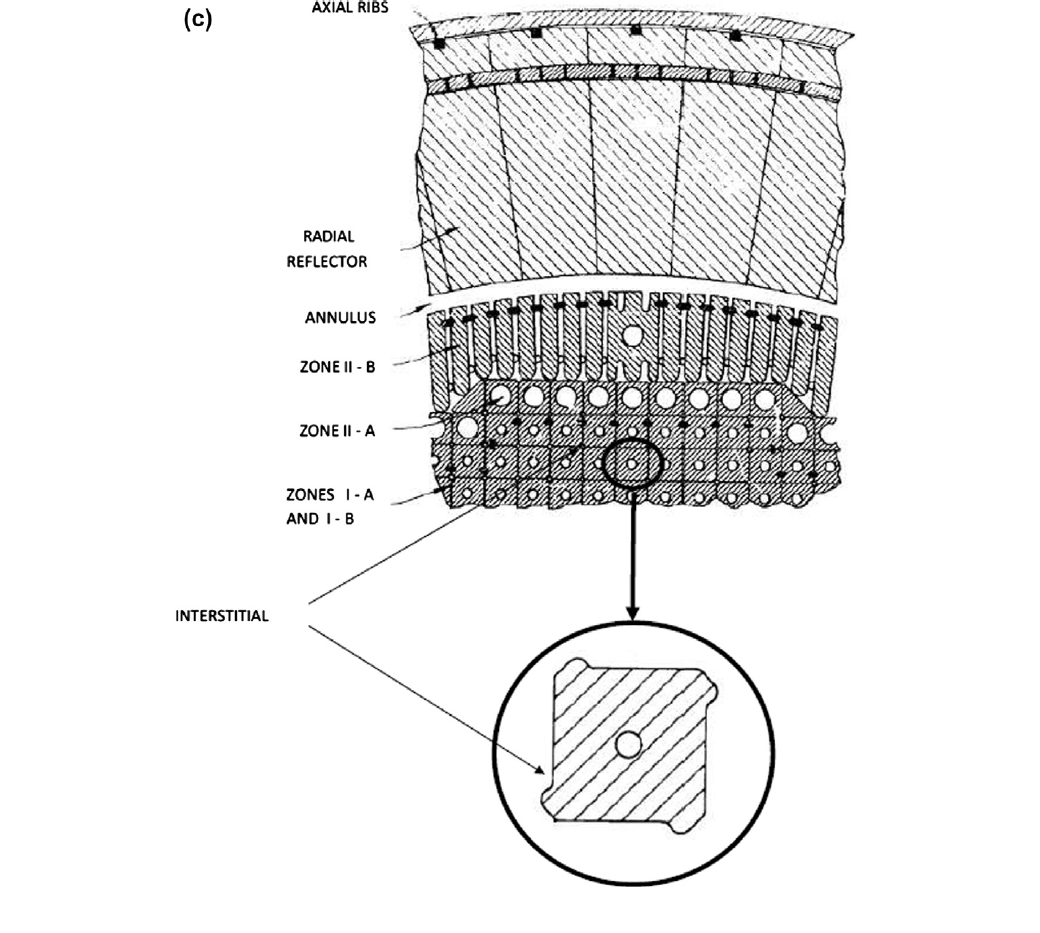(Robertson, 1979)

### MSBR Full Core Simulation

Andrei Rykhlevskii### MSBR Full Core Simulation

Andrei Rykhlevskii### MSBR Full Core Simulation

Andrei Rykhlevskii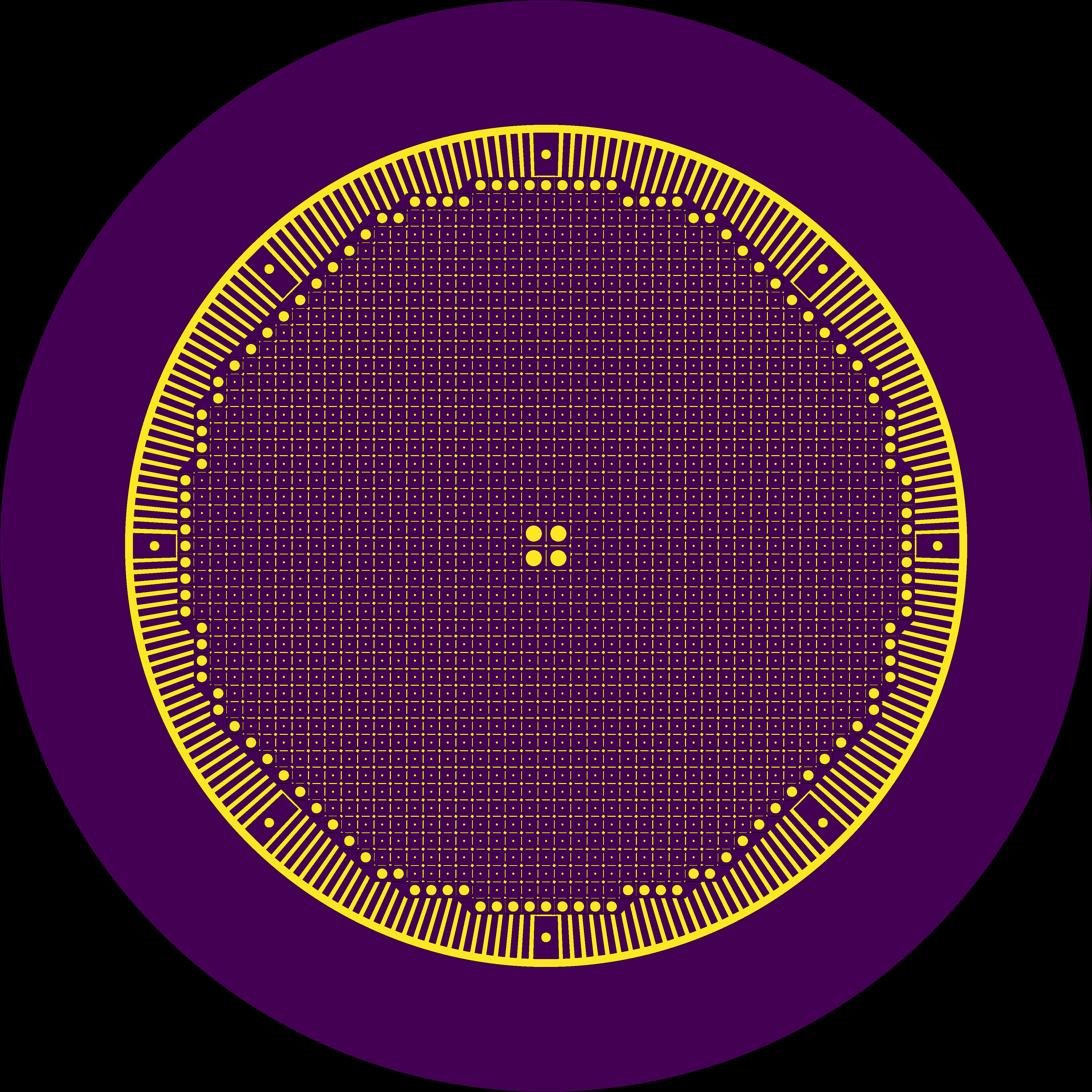### MSBR Full Core Simulation

Andrei Rykhlevskii### MSBR Full Core Simulation

Andrei Rykhlevskii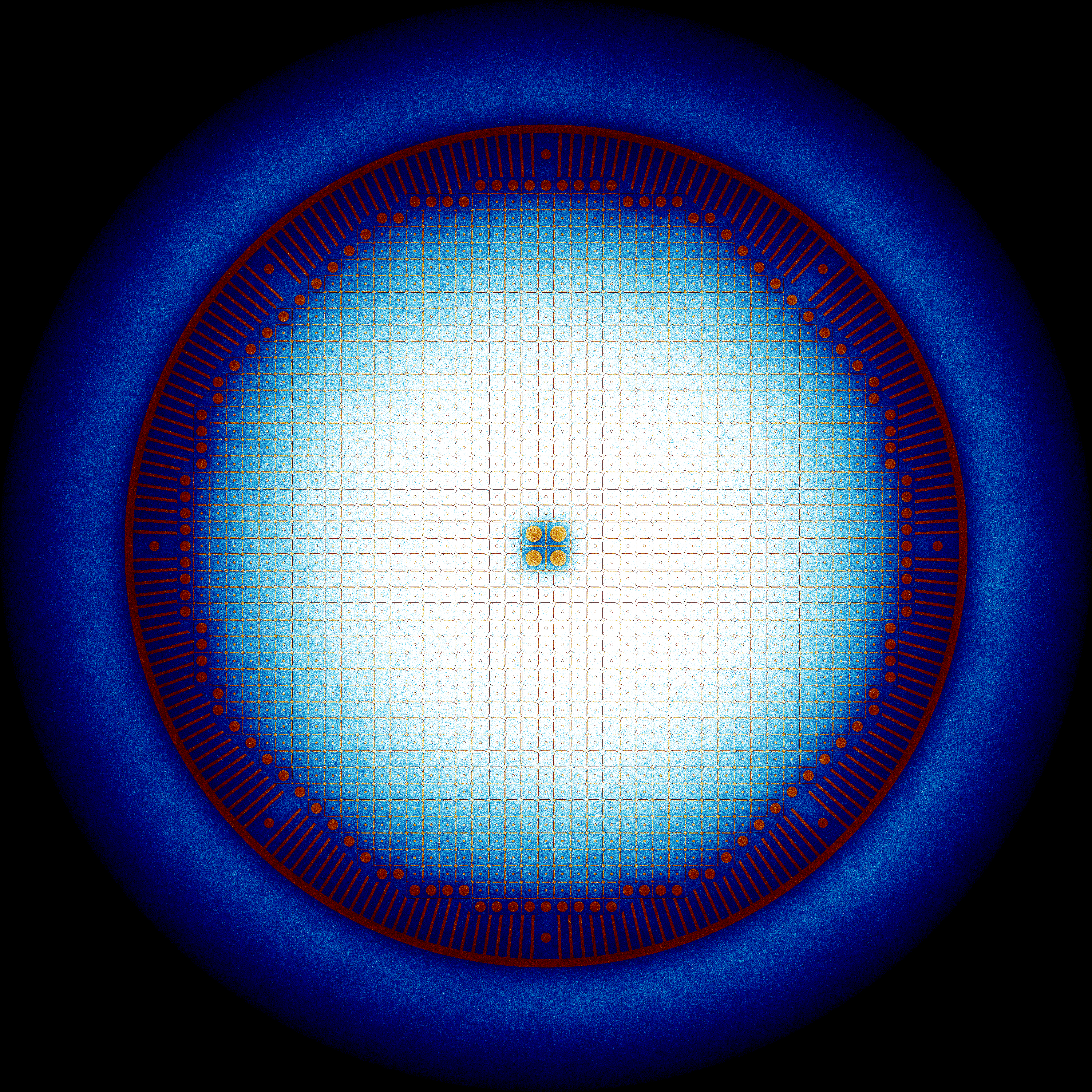### MSBR Full Core Simulation

Andrei Rykhlevskii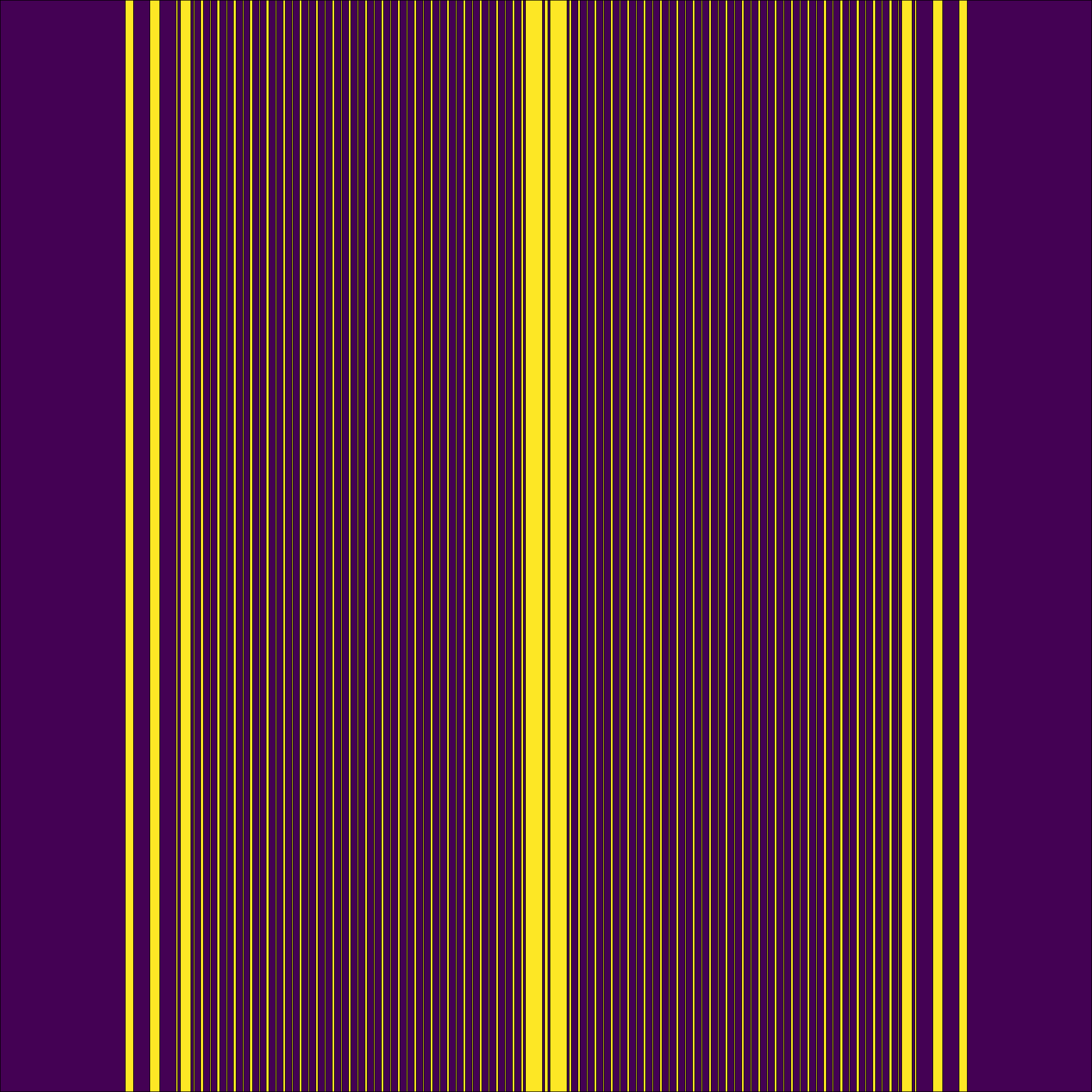### MSBR Full Core Simulation

Andrei Rykhlevskii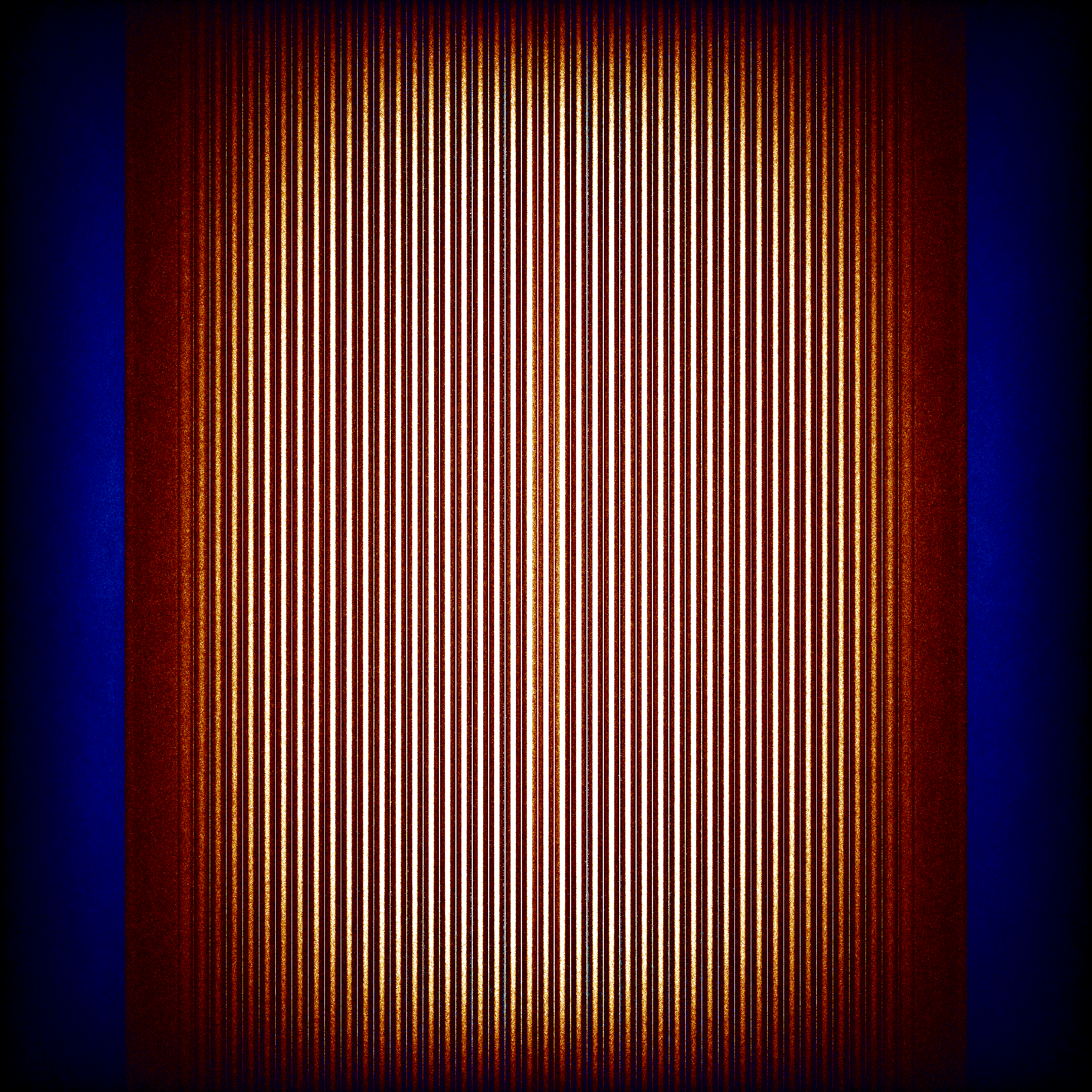### MSBR Full Core Simulation

Andrei Rykhlevskii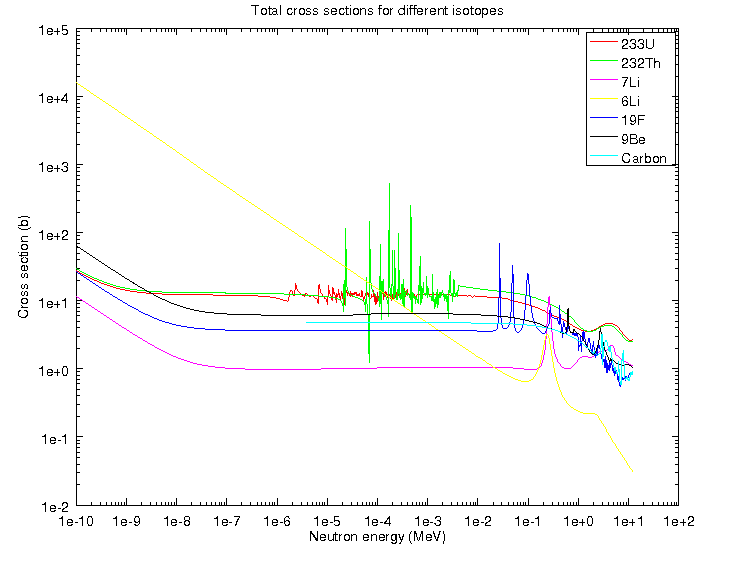### MSBR Full Core Simulation

Andrei Rykhlevskii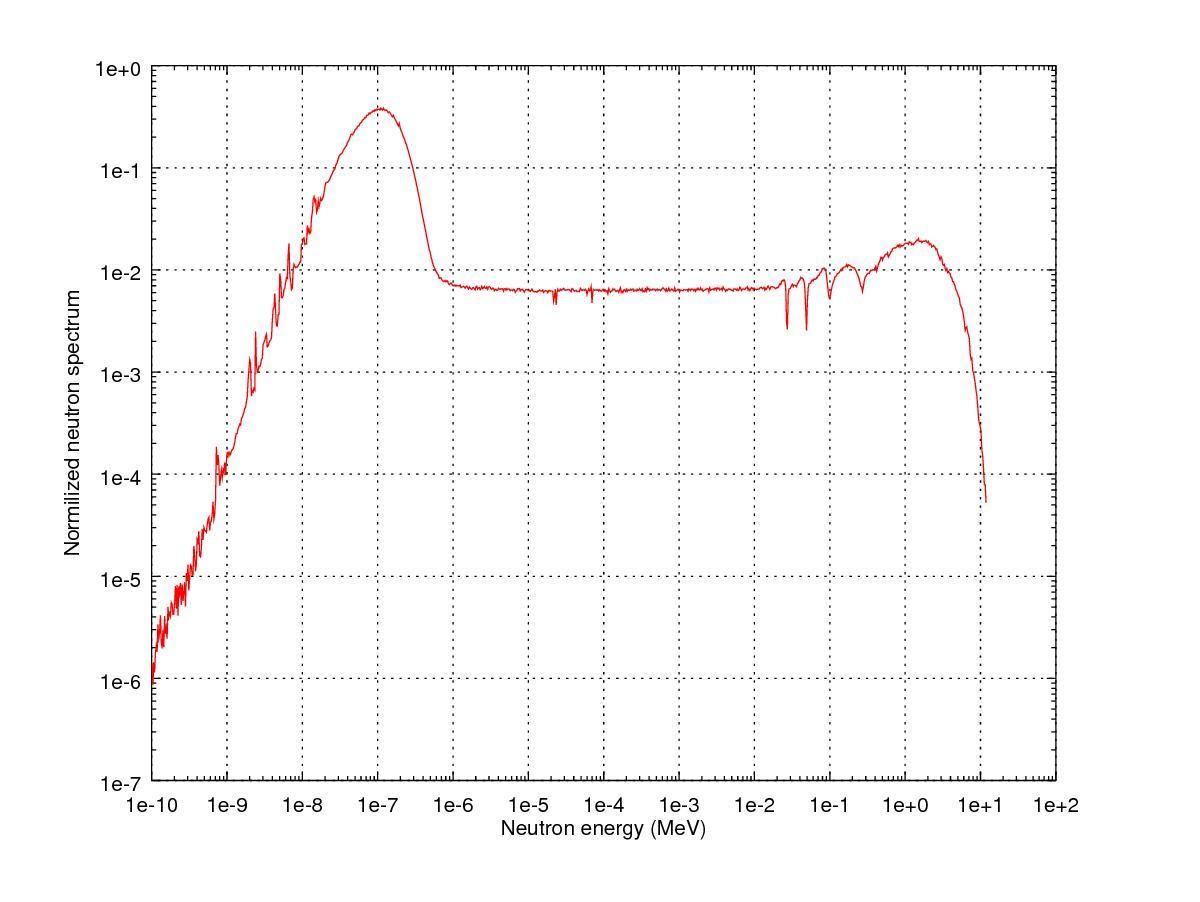## Reactor Kinetics$\sigma(E,\vec{r},\hat{\Omega},T,x,i)$$k=1$

## Reactivity

\begin{align} k &= \mbox{"neutron multiplication factor"}\\ &= \frac{\mbox{neutrons causing fission}}{\mbox{neutrons produced by fission}}\\ \rho &= \frac{k-1}{k}\\ \rho &= \mbox{reactivity}\\ \end{align}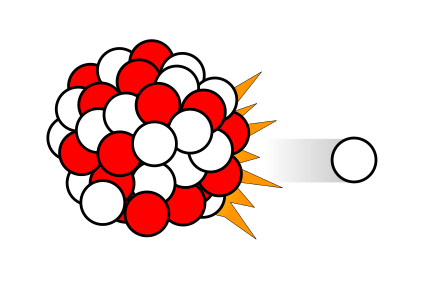$\beta_i, \lambda_{d,i}$

## Point Reactor Kinetics

\begin{align} p &= \mbox{ reactor power }\\ \rho(t,&T_{fuel},T_{cool},T_{mod}, T_{refl}) = \mbox{ reactivity}\\ \beta &= \mbox{ fraction of neutrons that are delayed}\\ \beta_j &= \mbox{ fraction of delayed neutrons from precursor group j}\\ \zeta_j &= \mbox{ concentration of precursors of group j}\\ \lambda_{d,j} &= \mbox{ decay constant of precursor group j}\\ \Lambda &= \mbox{ mean generation time }\\ \omega_k &= \mbox{ decay heat from FP group k}\\ \kappa_k &= \mbox{ heat per fission for decay FP group k}\\ \lambda_{FP,k} &= \mbox{ decay constant for decay FP group k}\\ T_i &= \mbox{ temperature of component i} \end{align}
$\frac{d}{dt}\left[ \begin{array}{c} p\\ \zeta_1\\ .\\ \zeta_j\\ .\\ \zeta_J\\ \omega_1\\ .\\ \omega_k\\ .\\ \omega_K\\ T_{i}\\ .\\ T_{I}\\ \end{array} \right] = \left[ \begin{array}{ c } \frac{\rho(t,T_{i},\cdots)-\beta}{\Lambda}p + \displaystyle\sum^{j=J}_{j=1}\lambda_{d,j}\zeta_j\\ \frac{\beta_1}{\Lambda} p - \lambda_{d,1}\zeta_1\\ .\\ \frac{\beta_j}{\Lambda}p-\lambda_{d,j}\zeta_j\\ .\\ \frac{\beta_J}{\Lambda}p-\lambda_{d,J}\zeta_J\\ \kappa_1p - \lambda_{FP,1}\omega_1\\ .\\ \kappa_kp - \lambda_{FP,k}\omega_k\\ .\\ \kappa_{k p} - \lambda_{FP,k}\omega_{k}\\ f_{i}(p, C_{p,i}, T_{i}, \cdots)\\ .\\ f_{I}(p, C_{p,I}, T_{I}, \cdots)\\ \end{array} \right]$

## Coupled Multi-Physics Analysis

Using the MOOSE framework and its Jacobian-Free Newton Krylov solver, severe accident neutronics and thermal hydraulics can be simulated beautifully for simple geometries and well studied materials. (below, INL BISON work.)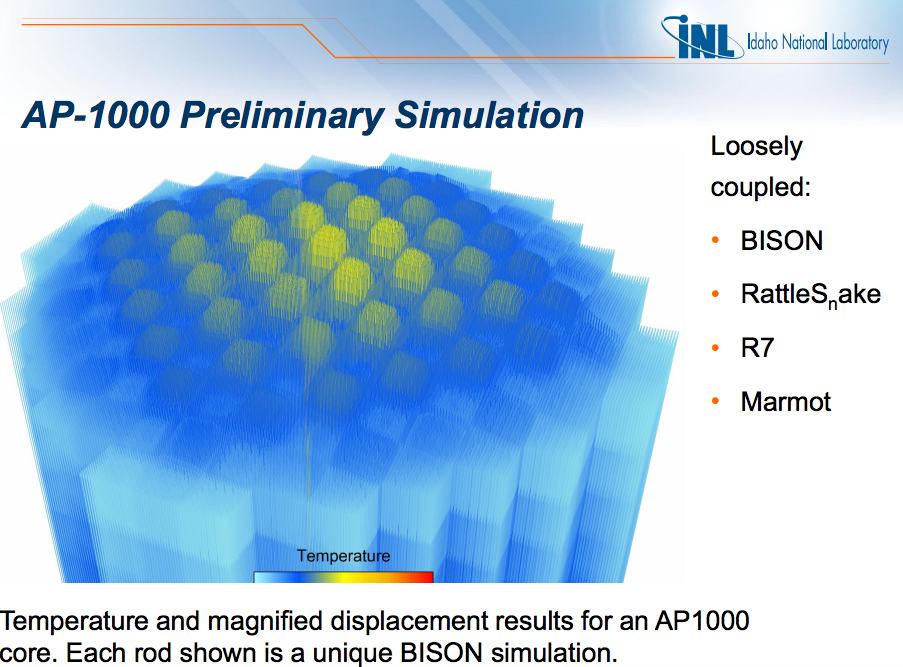## Moltres: Dr. Alexander Lindsay

### Moltres Current Capabilities

• Navier Stokes + Multi-Group Neutron Diffusion + Kinetics
• arbitrary number of neutron energy groups
• arbitrary number of delayed neutron precursor groups
• neutron power coupled to salt temperature and flow
• Precursor capability requires discontinuous galerkin upwind scheme.
• Few group constants can be generated with SCALE-NEWT or Serpent

## How Do We Know It's Right?## The Data Dilemma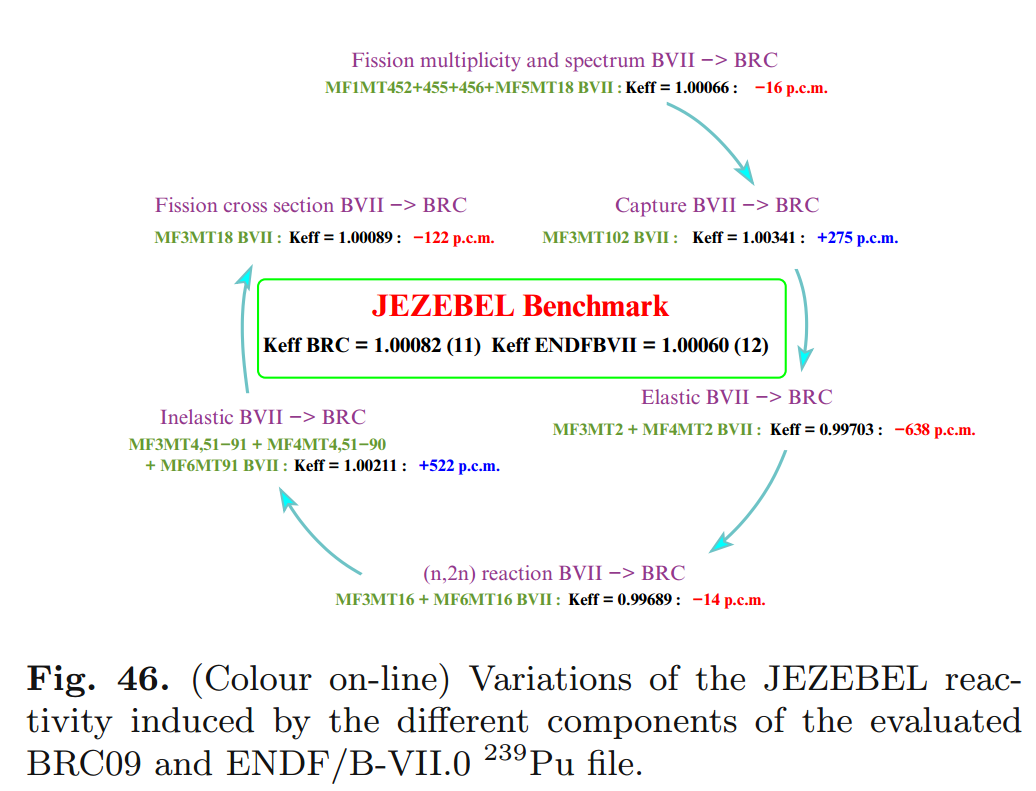## Unique Issues

Export control is serious.

# THE END

### Katy Huff

katyhuff.github.io/2017-05-31-pi4Based on a work at http://katyhuff.github.io/2017-05-31-pi4.

## A Few of My Favorite Things

• C++, Python, Fortran
• xml, markdown, rst, $\LaTeX$
• Doxygen, sphinx
• CMake, conda, macports